Maths-
General
Easy

Question

# Arrange the following rational numbers in descending order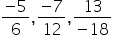and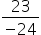.Hint:

## The correct answer is: Yes it's rational numbers

### Given rational numbers areand.Express the rational numbers with positive denominator by multiplying with.Here,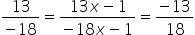and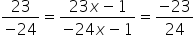So, Now we have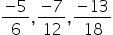and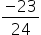.By taking LCM of denominators 6,12,18 and 24, we get LCM as 72On multiplying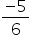with 12, we get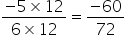On multiplying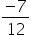with 6, we get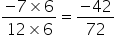On multiplying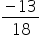with 4, we get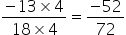On multiplyingwith 3, we get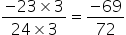On comparing the above fractions with same denominator,we have  -42 > -52 > -60 > -69Hence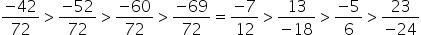So descending order is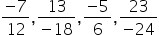#### With Turito Foundation.#### Get an Expert Advice From Turito.